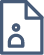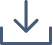### Recent Progress in Planar Curves

An enduring object of study of the field of geometric analysis is the relationship between the local and global properties of a shape. For example, if we seek to minimise the area of a surface bounding a given volume (a global property), is the boundary always a given by surface of constant positive curvature (a local property)? This is known as the isoperimetric problem, and has been studied for many centuries. The global property is often expressed as either an integral constraint, or else minimisation of an energy. This gives rise to local properties, often in the form of a differential equation that must be satisfied. The simplest of such geometric shapes is a closed curve in the plane. Remarkably, planar curves are still a rich field of study, despite their long history. One recent result is the fourvertex theorem. This states that the curvature of a closed curve must have four extremal points. The converse to this was only discovered in 1998, by Dahlberg. Another example is the knotting of curves: is there a local or global property that will detect whether a curve is knotted?#### Jia Jia Qian

Monash University

Jia Jia is a third-year undergraduate student studying maths and computer science student at Monash University. She is interested in graph theory and analysis, and hopes to explore many more areas of mathematics.